Although these can be used for maps between the surfaces the intended purpose is mapping the volume. I’ll make no comments on reductions for that case. This is going to be even terser than the square/disc post and I’ll skip on things like showing the Jacobian and comments about adding bias to remove degenerate regions (or even that they exist).

I will use the convention that $\left(x,~y,~z\right)$ is a coordinate in the cube and $\left(u,~v,~w \right)$ a coordinate in the ball.

In this post I’ll normalize the volume distortion values for the plots. The volume of cube is eight, the ball is $\frac{4}{3}\pi$, the Jacobian determine is multiplied by $\frac{6}{\pi}$ so the value of equal volume is one.

Define a signum like function:

$\text{sgn}\left(x\right) = \begin{cases} 1 & x \geq 0 \\[2ex] -1 & x < 0 \end{cases}$

Proof-of-concept C code here.

As with square/disc the most straight forward map is radial stretch. Cube to ball:

$\left( u,~v,~w \right) = \frac{\text{max}\left( \abs{x},~\abs{y},~\abs{z} \right)}{\sqrt{x^2+y^2+z^2}}\left(x,~y,~z\right)$

The Jacobian determinate:

$\frac{ \max \left(\abs{x},~\abs{y},~\abs{z}\right)^3}{\left(x^2+y^2+z^2\right)^{\frac{3}{2}}}$

The volume distortion (determinate multiplied by $\frac{6}{\pi}$):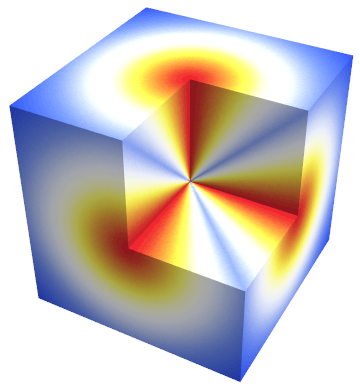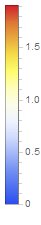Ball to cube:

$\left( x,~y,~z \right) = \frac{\sqrt{u^2+v^2+w^2}}{\text{max}\left( \abs{u},~\abs{v},~\abs{w} \right)}\left(u,~v,~w\right)$

## Fong cube/ball

Fong1 derives a number of 3D blending equations as an extension of his 2D work.

One of which is a cube to ball:

$\left( u,~v,~w \right) = \frac{\sqrt{x^2+y^2+z^2-\left(x^2y^2+y^2z^2+x^2z^2\right)+x^2 y^2 z^2}}{\sqrt{x^2+y^2+z^2}}\left(x,~y,~z\right)$

We could rewrite the numerator a couple of way that drops the additional products to three. The Jacobian determinate:

$\frac{\sqrt{x^2 \left(y^2-1\right) \left(z^2-1\right)-y^2 \left(z^2-1\right)+z^2} \left(x^2 \left(y^2 \left(3 z^2-2\right)-2 z^2+1\right)+y^2 \left(1-2 z^2\right)+z^2\right)}{\left(x^2+y^2+z^2\right)^{\frac{3}{2}}}$

The volume distortion: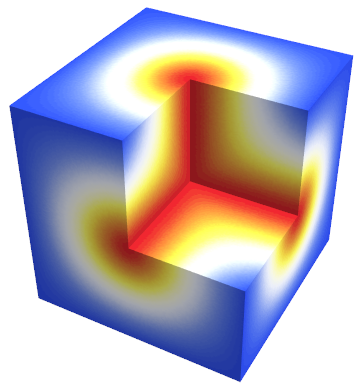Fong derives the ball to cube map, however it involves solving a cubic so I’ll give it a pass for now.

## Nowell cube/ball

Nowell in a blog post2 presented a cube to ball map:

$\left( u,~v,~w \right) = \left( x \sqrt{1-\frac{1}{2}y^2- z^2\left(\frac{1}{2} + \frac{1}{3}y^2 \right)}, ~y \sqrt{1-\frac{1}{2}z^2- x^2\left(\frac{1}{2} + \frac{1}{3}z^2 \right)}, ~z \sqrt{1-\frac{1}{2}x^2- y^2\left(\frac{1}{2} + \frac{1}{3}x^2 \right)} \right)$

The volume distortion: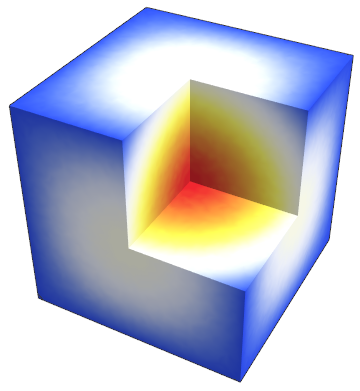As far as I know nobody has bothered to derive the inverse function3. The density distribution could make worthwhile to solve at some point, but not today.

## Volume preserving cylinder/ball bi-Lipschitz

A paper from 2008 4 derives a volume preserving cylinder to ball map (generalized to $n$-dimensions).

The volume preserving map of cylinder $\left(a,b,z\right)$ with $z \in \left[-1,1\right]$ and a radius of one to ball:

$\left( u,~v,~w \right) = \begin{cases} \left(a \sqrt{\frac{2}{3}-\frac{a^2+b^2}{9 z^2}}, b \sqrt{\frac{2}{3}-\frac{a^2+b^2}{9 z^2}}, z-\frac{a^2+b^2}{3 z} \right) & z^2 \geq a^2+b^2 \\[2ex] \left(a \sqrt{1-\frac{4 z^2}{9 \left(a^2+b^2\right)}}, b \sqrt{1-\frac{4 z^2}{9 \left(a^2+b^2\right)}}, \frac{2}{3}z \right) & z^2 \leq a^2+b^2 \end{cases}$

The cases correspond to points inside and outside of the embedded conic respectively.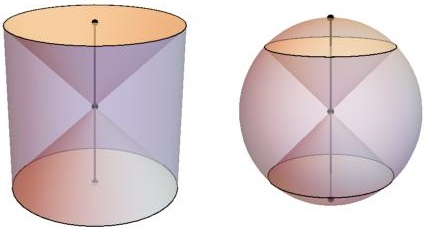The ball to cylinder map into two steps. First a common term $t$, a scale factor $s$ and compute $z$:

$t = \sqrt{u^2+v^2+w^2}$ $\left(s, ~z\right) = \begin{cases} \left( \sqrt{3\frac{t}{t+\abs{w}}}, ~\text{sgn}\left(w\right) t \right) & \frac{5}{4}w^2 > u^2+v^2 \\[2ex] \left( \frac{t}{\sqrt{u^2+v^2}}, ~\frac{3}{2}w \right) & \frac{5}{4}w^2 \leq u^2+v^2 \end{cases}$

Then the cylinder can be expressed as:

$\left(a, ~b, ~z\right) = \left(su,~sv,~z \right)$

## As many cube/cylinder/ball maps as you can shake a disc at

Given the volume preserving cylinder/ball map (let’s call it $L$ and its inverse $L^{-1}$) we can construct a cube/ball from any square/disc mapping. If $D$ is a square to disc map and $D^{-1}$ the inverse, then the cube to ball map is:

$\left( u,~v,~w \right) = L(D(x,~y),~z)$

And the ball to cube map:

$\left( x,~y,~z \right) = L^{-1}(D^{-1}(u,~v),~w)$

The volume distortion of the resulting map is simply (rescaled) area distortion of $D$ for all $z$ slices since $L$ preserves volume. I won’t bother with any more equations. Although redundant I will show the volume distortions for the various disc methods. The gradients are not ideal since these are not mapping one to white, but they all consistent.

### Volume preserving cube/ball

The same paper as cylinder/ball gives the concentric disc method to complete a volume preserving cube/ball map.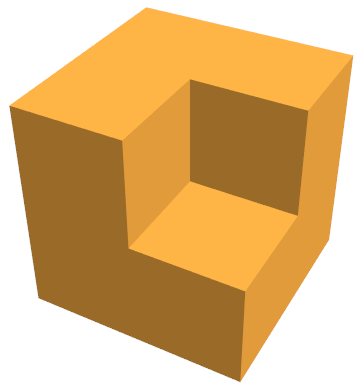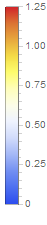### Approximate volume preserving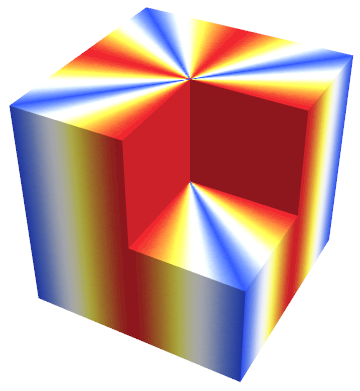### Disc squircle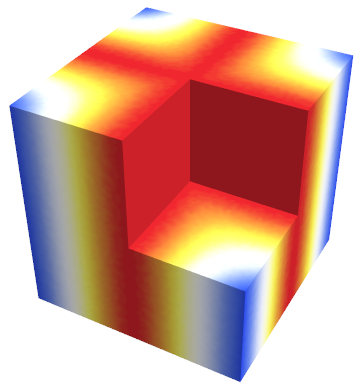### Disc elliptical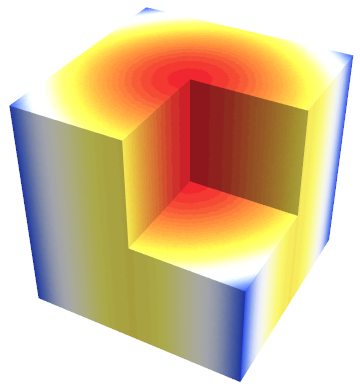## References and Footnotes

1. “Squircular Calculations”, Chamberlain Fong, 2015. (arXiv

2. “Mapping a Cube to a Sphere”, Philip Nowell, 2005. (link

3. A specical case inverse of sphere to cube surface can be found here: (link

4. “A bi-Lipschitz continuous, volume preserving map from the unit ball onto a cube”, Griepentrog, Hoppner, Kaiser, Rehberg, 2008 (PDF